# How to calculate time difference between two times or dates?

When you have two lists of times, such as column A and column B, and the time in column A is earlier than the time in column B in the same row, but you are unable to determine the correct time by subtracting the end time from the earlier one, then you have a problem. Now that we have this out of the way, let's discuss how to compute the time difference using Excel.

## Calculating the Time Difference Between Two Times in Excel

In this scenario, we are going to assume that there are just two columns containing the hours, minutes, and seconds, and that the time in column A is always going to be earlier than the time in column B in the same row.

Let’s understand step by step with an example.

### Step 1

In the first step, let us assume we have a sample data for calculating time difference between two times as shown in the below screenshot for the same.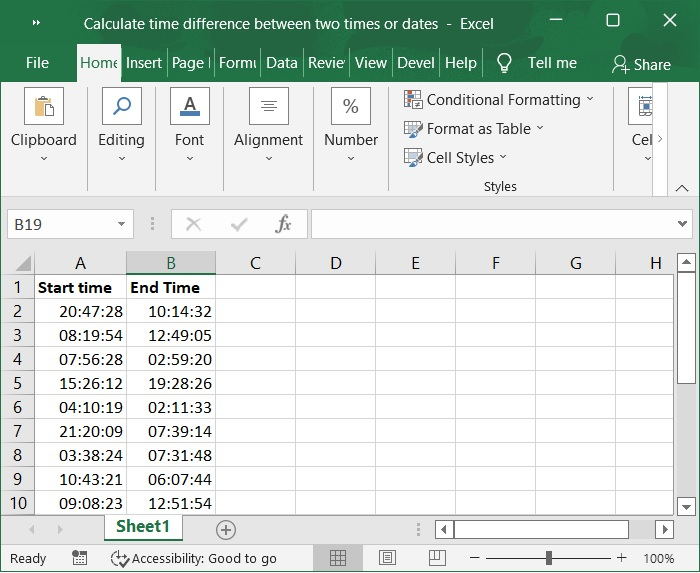### Step 2

Now, choose a cell that is empty. In this example, I choose Cell C2 and type in the following formula:

=B2-A2+(A2>B2)


Here, cell A2 displays a time that is start time and Cell B2 is end time, however you can switch the times around to suit your needs. Please refer to the below screenshot for the same.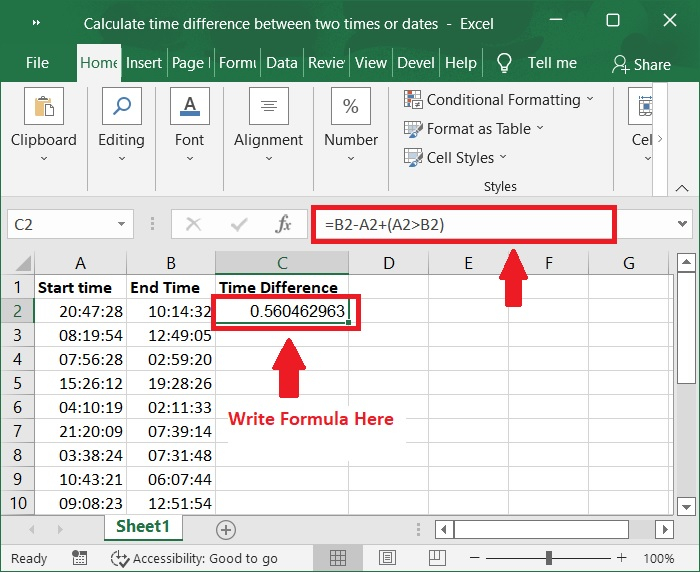### Step 3

After that, press the Enter key, you can then drag the fill handle to fill the range that is required for this formula to work. Please check out the below screenshot for the same.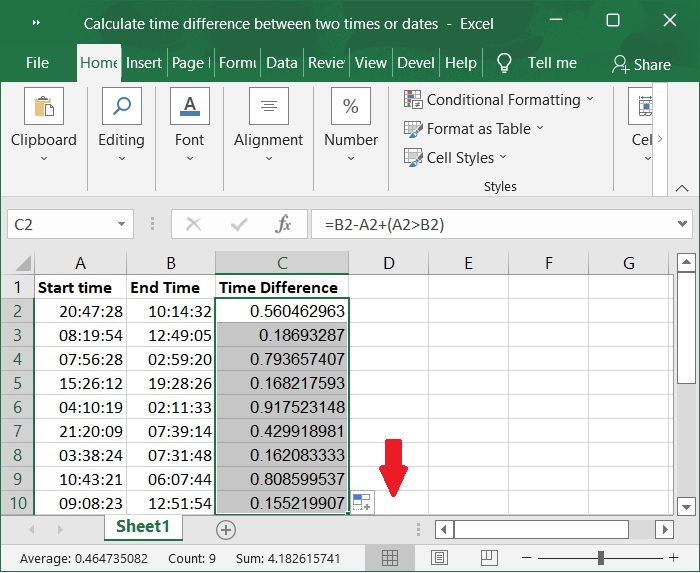### Step 4

In, the next step is to convert the numerical values into time format. Right click on the result range, in this case the range C2:C10, and then select Format Cells from the context menu when you press the right mouse button it will appeared. Below screenshot for the same.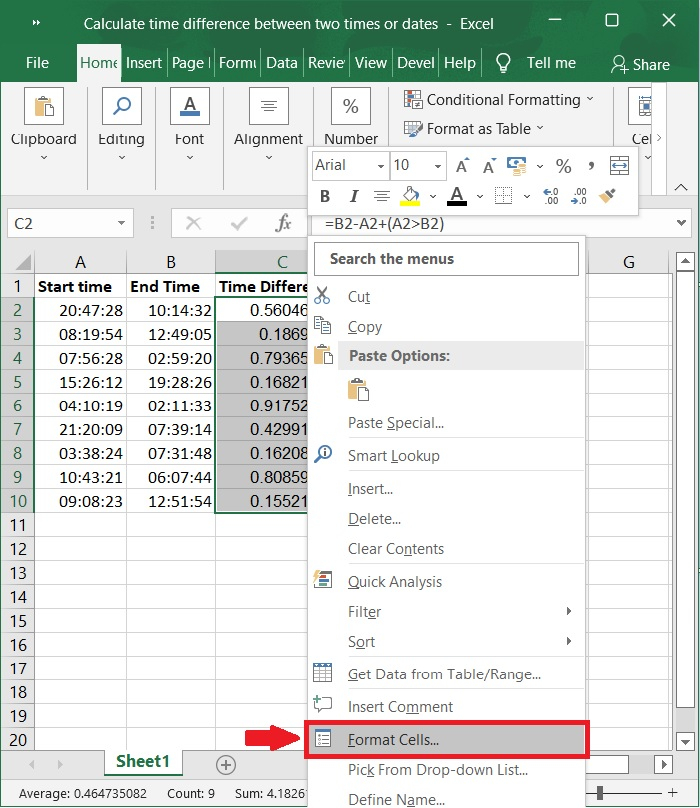### Step 5

Then, the Format Cells dialog box displayed, choose the Number tab, then click Time. Then, in the right-hand area, select the time format that you require, and then click OK button to close the dialog box. Please check out below screenshot for the same.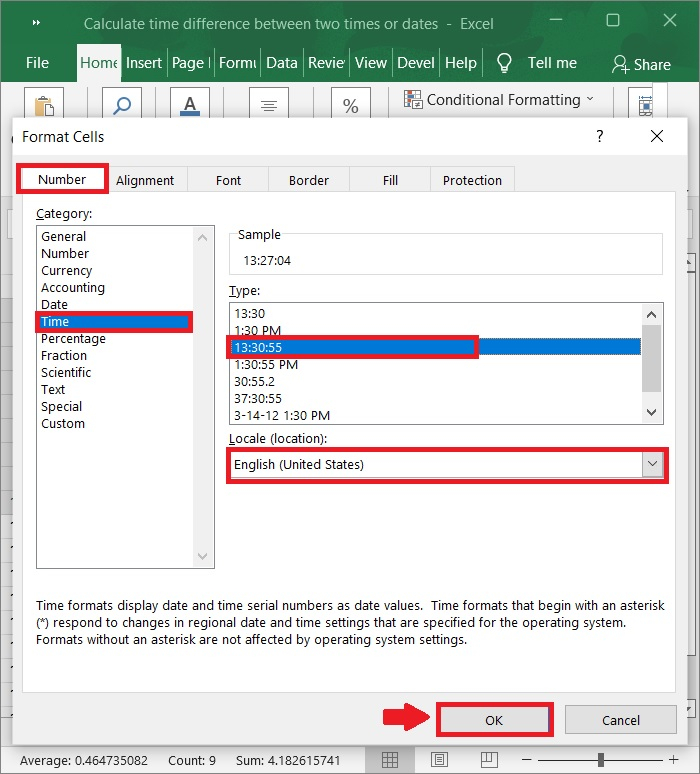### Step 6

In addition, the numerical values have been changed and displayed in time format. Please refer to the below screenshot for the same.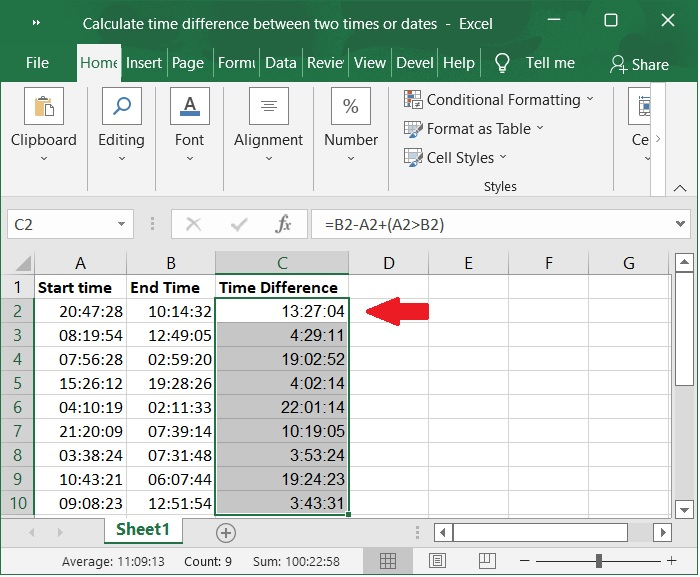Important Note − If you want to calculate time difference between two dates, you can use this formula and then use format cell to format numerical values into time format.

=B2-A2


## Conclusion

In this tutorial, we explained how you can use the features in Excel to calculate the time difference between two times by using formula and "Format Cells" option.

Updated on: 06-Feb-2023

450 Views